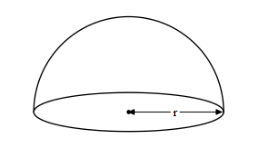# Area of the base of a solid hemisphere is $36\pi$ sq. cm. Then its volume is(a) $288\pi \,c{{m}^{3}}$ (b) $108\pi \,c{{m}^{3}}$ (c) $144\pi \,c{{m}^{3}}$ (d) $72\pi \,c{{m}^{3}}$Verified
204.3k+ views
Hint: In order to solve this question, we need to understand that the radius of the sphere is itself the radius of the base of the hemisphere. Therefore, we need to find the radius with the help of the area of the base. Later, with the help of the radius, we can find the volume of the hemisphere. The formula for the volume of the hemisphere is given by $\text{Volume}=\dfrac{2}{3}\pi {{r}^{3}}$, where r is the radius of the hemisphere.

We are given the base of the semicircle and we need to draw the volume of the hemisphere.
We need to understand the question with the help of the diagram.Let the radius of the hemisphere by r.
As we are given the area of the base of the hemisphere, we can find the radius of the hemisphere.
The area of the circle interns of the radius is $Area=\pi {{r}^{2}}$.
Substituting the values of the area we get,
$36\pi =\pi {{r}^{2}}$
Solving the equation for r we get,
${{r}^{2}}=36$
Taking the square root, we get,
$r=6$ cm.
Now, with the help of the radius, we need to find the volume of the hemisphere.
The formula for the volume of the hemisphere is as follows,
$\text{Volume}=\dfrac{2}{3}\pi {{r}^{3}}$
Substituting the value of r we get,
$\text{Volume}=\dfrac{2}{3}\pi \times {{6}^{3}}$
Solving this we get,
\begin{align} & \text{Volume}=\dfrac{2}{3}\pi \times {{6}^{3}} \\ & =\dfrac{2\times 6\times 6\times 6}{3}\times \pi \\ & =4\times 36\times \pi \\ & =144\pi \,c{{m}^{3}} \\ \end{align}
Therefore, the volume of a solid hemisphere is $144\pi \,c{{m}^{3}}$ .
So, the correct answer is “Option C”.

Note: In this problem, we can remember the formula of hemisphere very easily. We know the hemisphere is just a sphere cut into half So the volume of the sphere is also divided into half. Therefore, we can say that the Volume of hemisphere = half of the volume of the sphere. Hence, once we know the volume of the sphere, we can easily remember the volume of the hemisphere.